blog | How to Calculate RMS Torque |
|

##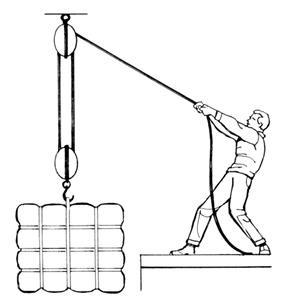Block and Tackle Series Volume 3 – How to Calculate RMS Torque

Question:

How do I calculate RMS (Root Mean Square) Torque for a given axis motion profile in my application?

In our last Block and Tackle posting, we touched on operating a motor in a hotter ambient temperature. For this posting, we take a look at the Root Mean Square (RMS) Torque and why it is important. Typically an axes’ motion profile is broken up into multiple segments, each segment is found to require a specific torque for a specific amount of time to complete the desired motion. For example, this can include torque required to accelerate, traverse (against an external force and/or friction), decelerate, and dwell. Each of these segments affects the amount of heating the motor experiences and thus the equivalent steady state continuous requirement utilized to select the correct motor. The RMS Torque calculation considers not only the amount of torque, but also the duration of that torque (by segment). Our example below illustrates how to calculate Trms of your motion profile.

The below motion profile would be broken up into eight (8) different segments, each with a required torque Tx and time tx.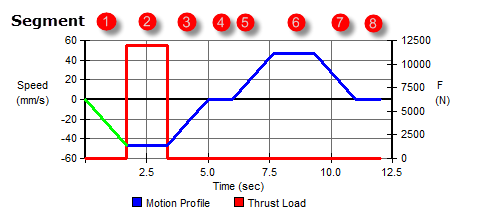To calculate Trms, use this equation: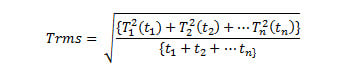Where T1 = torque required by and during segment 1, and t1 = time duration (t1-t0) of segment 1, etc.. Note the additional torque required by the motor to over come some external/thrust force (greater than friction alone) during segment 2, and the lack of this required torque during the dwell segment 4 and 8.

Going back to the example motion profile above and the chart of that motion profile: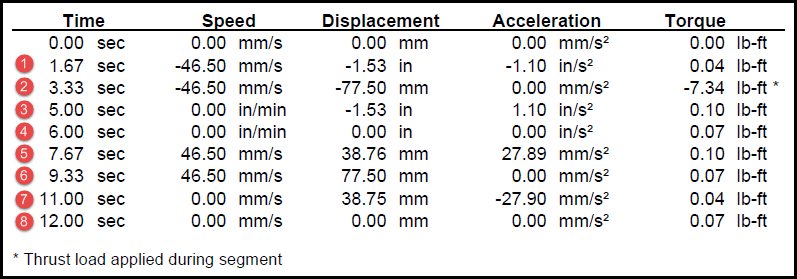Therefore, if you do the math – and we will spare you writing this into a very long equation, the result is:

Trms =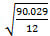= 2.74 lb-ft or 3.715_Nm (1.35582_Nm/lb-ft.)